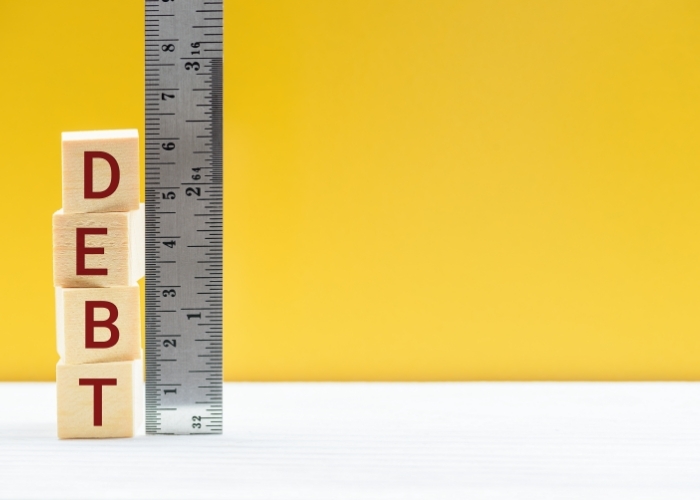# Debt Yield: Real Estate Definition & Why It’s Important#### IN THIS GUIDE

Debt yield is a key metric that lenders use to determine how long it would take to recoup losses in the case of borrower default. It is calculated by dividing the net operating income generated by a property by the total amount of debt used to purchase that property. This figure allows lenders to evaluate borrowers and make sure they’re reducing risk as much as possible.

Real estate investors who understand how debt yield is calculated—and why it is important—can better anticipate their approval odds for commercial real estate financing. Likewise, debt yield can help investors compare commercial real estate to find the most productive properties.

## What Is Debt Yield in Real Estate?

Debt yield lets commercial real estate lenders determine the risk posed by a loan based on how quickly it could recoup its losses in case of borrower default. It is calculated by dividing the annual income generated by a property by the total amount financed.

Debt yield is important because it helps lenders calculate how long it would take a property to generate sufficient income to recoup an outstanding loan balance. In this way, the figure allows lenders to assess the risk of a loan and make sure they are not over-leveraging themselves.

## What Is a Good Debt Yield?

A low debt yield means that a property is not generating enough income to cover the loan payments. A good debt yield should be at least 10%, but the higher the percentage, the safer the loan is in the eyes of the lender. Anything lower and the lender may be unwilling to finance the property.

## Debt Yield Calculation

To calculate debt yield, you will need the following information:

• The purchase price of the property
• The down payment amount
• The annual income generated by the property

Once you have this information, plug it into the following formula:
Debt Yield = Annual Income / (Purchase Price – Down Payment) x 100

### Debt Yield Example

Consider a commercial property that costs \$2,000,000 and a real estate investor who plans to put 20% down (\$400,000). The annual income generated by the property is \$100,000.

Using the formula above, we can calculate the debt yield as follows:

Debt Yield = \$100,000 / (\$2,000,000 – \$400,000) x 100

Debt Yield = 6.25%

As you can see, the debt yield is 6.25%. This is a low debt yield and means that a lender is not likely to extend financing.

Alternatively, consider a real estate investor who wants to purchase a \$2,000,000 property by putting the same percentage down as in the previous example. In this case, however, the annual income generated by the property is \$200,000.

The calculation under this scenario is as follows:

Debt Yield = \$200,000 / (\$2,000,000 – \$400,000) x 100

Debt Yield = 12.5%

## Other Lender Criteria

When evaluating the risk of financing a property, lenders look at several factors beyond just debt yield. Consider these metrics when considering your next real estate investment:

### Loan-to-Value (LTV)

Loan-to-value (LTV) ratio is another important metric that lenders use to assess the risk of a loan. The LTV ratio is calculated by dividing the loan amount by the purchase price of the property. Lenders typically like to see an LTV ratio of 80% or less. If the LTV is higher, a lender may not approve the loan.

LTV = Loan Amount / Purchase Price

For example, if you are looking at a property that costs \$100,000 and you have a \$20,000 down payment, your loan amount would be \$80,000. This would give you an LTV ratio of 80%.

### Debt Service Coverage Ratio (DSCR)

The debt service coverage ratio (DSCR) is also used by lenders to assess the risk of a loan. To calculate DSCR, divide the net operating income (NOI) of a property by the total amount of debt payments.

DSCR = Net Operating Income / Debt Payments

Lenders typically prefer a DSCR of at least 1.15 to 1.25, which means that the property is generating enough income to slightly more than cover the debt payments. However, a DSCR of at least 1.5 is preferable. A DSCR of less than 1 means the income is only enough to make a portion of the monthly payment.

Consider a property that generates \$12,000 in net operating income with monthly debt payments of \$2,000. In this case, the DSCR is 12 (a very strong DSCR), and the loan is likely to be approved by most lenders.

### Cap Rate

A property’s capitalization rate—or cap rate—represents a real estate investment’s rate of return, expressed as a percentage. The cap rate of a property is calculated by dividing the NOI by the current market value of the property. This formula can be modified to instead include the acquisition price of the property by dividing NOI by the purchase price. However, this is a less accurate representation of the rate of return.

Cap Rate = Net Operating Income / Current Market Value

A cap rate between 7% and 10% is generally considered good, with cap rates over 10% often indicating a less rent-stable property. For example, a commercial property that generates \$12,000 in NOI and costs \$100,000 to purchase, has a cap rate of 12%. Depending on the investor’s risk tolerance, this may be too high—as a high cap rate is usually due to unstable income.